fanimator

Create stop-motion animation object

Description

example

fanimator(f) creates a stop-motion animation object from the function f. The function f must return graphics objects that depend on only one variable. This variable defines the time parameter of the animation.

By default, fanimator creates stop-motion frames of f(t0), generating 10 frames per unit interval of t0 within the range of t0 from 0 to 10.

example

fanimator(f,args) allows the function f to depend on multiple variables. args specifies the input arguments of f.

By default, the variable t = sym('t') is the time parameter of the animation. This syntax creates stop-motion frames of f(subs(args,t,t0)) within the range of t0 from 0 to 10. You can animate a specific property of the graphics objects by setting its value to depend on t in the input argument args.

example

fanimator(___,Name,Value) specifies the animation properties using one or more Name,Value pair arguments. Use this option with any of the input argument combinations in the previous syntaxes. Name-value pair settings apply to the animation object created.

example

fanimator(ax,___) creates a stop-motion animation object in the axis specified by ax instead of in the current axis (gca). The option ax can precede any input argument combinations in the previous syntaxes.

fp = fanimator(___) returns an Animator object. Use fp to query and modify the properties of a specific animation object. For a list of properties, see Animator Properties.

Examples

collapse all

Animate a point and a circle that move along a straight line.

First, create a function to plot a point at (t,1). The variable t defines the time parameter of the animation.

f = @(t) plot(t,1,'r*');

Create a stop-motion animation object defined by f.

fanimator(f)Next, create a function handle by using fplot to plot a unit circle. The circle is a function of two variables.

Create two symbolic variables t and x. Use t to set the center of the circle at (t,1) and x to parameterize the perimeter of the circle within the range [-pi pi]. Add the circle animation object to the existing plot. Set the x-axis and y-axis to be equal length.

syms t x
hold on
fanimator(@fplot,cos(x)+t,sin(x)+1,[-pi pi])
axis equal
hold off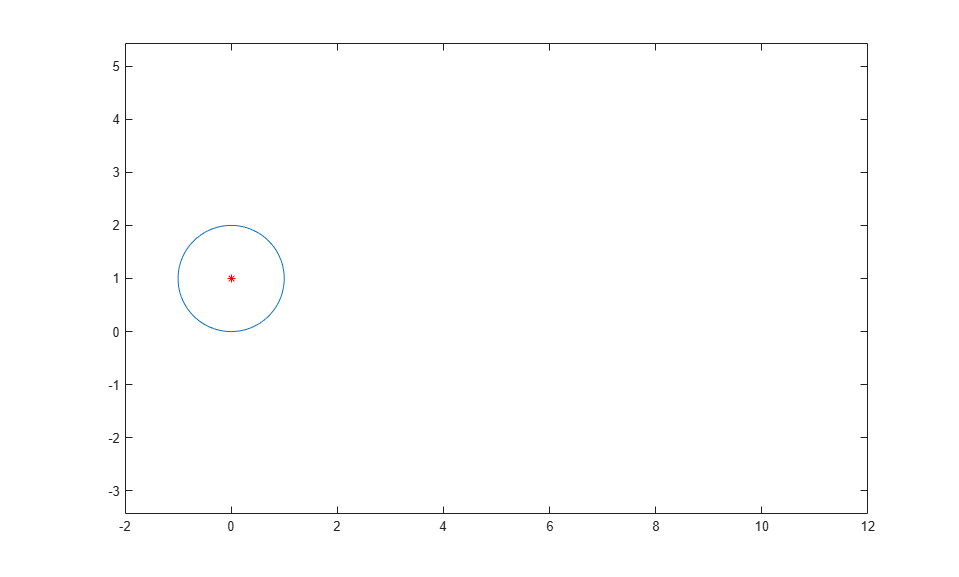Enter the command playAnimation to play the animation. By default, fanimator creates an animation object, generating 10 frames per unit time within the range of t from 0 to 10.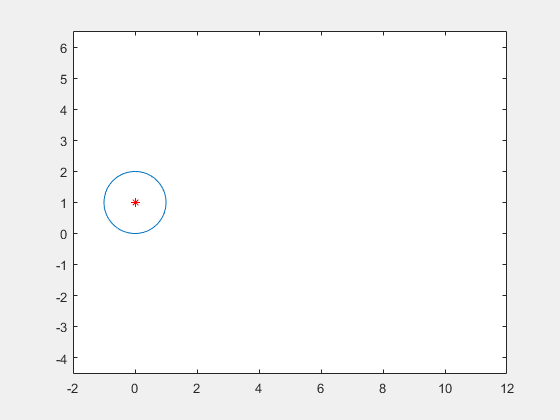Animate a line that changes vertical length and line width. You can animate a specific graphics property by setting its value to depend on the animation time parameter. By default, the variable t is the time parameter of the animation.

Create two symbolic variables, y and t. Plot a line with y coordinates within the interval [0 t] by using fplot. Use the fanimator function to create the line animation object. fanimator changes the line vertical length by increasing the value of t from 0 to 10.

syms y t
fanimator(@fplot,1,y,[0 t])Enter the command playAnimation to play the animation.Now plot a line with y coordinates within the interval [0 2] by using fplot. Set the 'LineWidth' property value to t+1. Use the fanimator function to create the line animation object. fanimator changes the line width by increasing the value of t from 0 to 10.

fanimator(@fplot,1,y,[0 2],'LineWidth',t+1)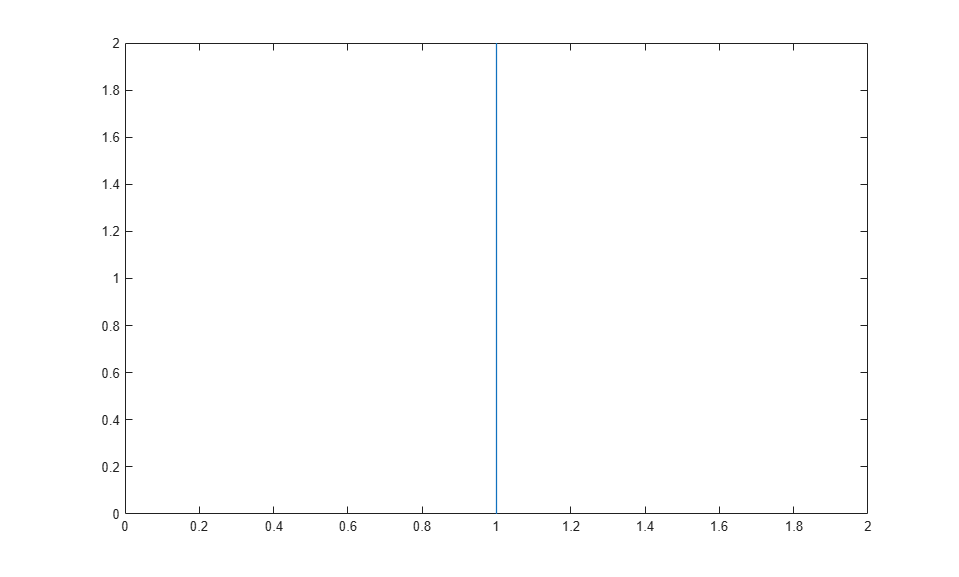Enter the command playAnimation to play the animation.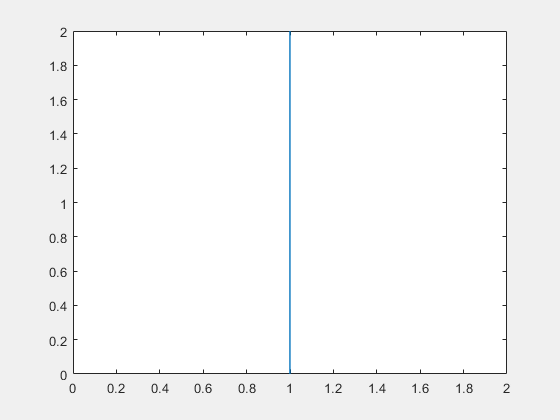Animate a circle with a timer.

First, create a function that plots a unit circle and save it in a file named circ.m. The function uses fplot to plot a unit circle centered at (t,1), and the local symbolic variable x to parameterize the perimeter of the circle.

function C = circ(t)
x = sym('x');
C = fplot(cos(x)+t,sin(x)+1,[0 2*pi],'Color','red');
end

Use fanimator to create a unit circle animation object. Set the animation range of the time parameter to [2 4.5] and the frame rate per unit time to 4. Set the x-axis and y-axis to be equal length.

fanimator(@circ,'AnimationRange',[2 4.5],'FrameRate',4)
axis equal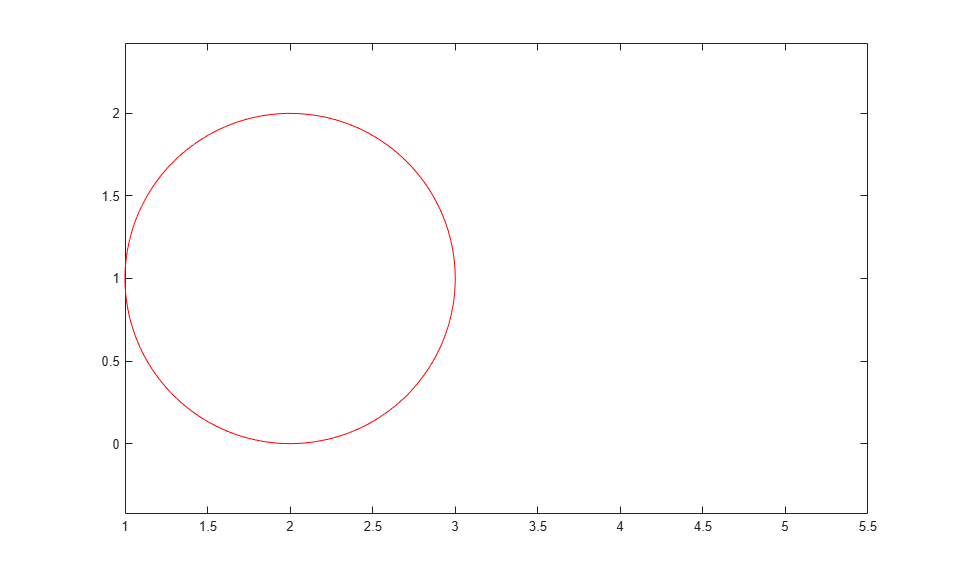Next, add a timer animation object. Create a piece of text to count the elapsed time by using the text function. Use num2str to convert the time parameter to a string. Set the animation range of the timer to [0 4.5].

hold on
fanimator(@(t) text(4.5,2.5,"Timer: "+num2str(t,2)),'AnimationRange',[0 4.5])
hold offEnter the command playAnimation to play the animation. The timer counts the elapsed time from 0 to 4.5 seconds. The moving circle starts at 2 seconds and stops at 4.5 seconds.Animate two cycloids in separate axes. A cycloid is the curve traced by a fixed point on a circle as the circle moves along a straight line without slipping.

First, create two symbolic variables x and t. Create a figure with two subplots and return the first axes object as ax1. Create a moving circle animation object in ax1 and add a fixed point on the rim of the circle. Set the x-axis and y-axis to be equal length.

syms x t
ax1 = subplot(2,1,1);
fanimator(ax1, @fplot, cos(x)+t, sin(x)+1, [-pi pi])
axis equal
hold on
fanimator(ax1, @(t) plot(t-sin(t), 1-cos(t), 'r*'))To trace the cycloid, use a time variable in the plotting interval. The fplot function plots a curve within the interval [0 t]. Create the cycloid animation object. By default, fanimator creates stop-motion frames within the range of t from 0 to 10 seconds. fanimator plots the first frame at t equal to 0.

fanimator(ax1, @fplot, x-sin(x), 1-cos(x), [0 t], 'k')
hold offNext, create another cycloid on the second axes object ax2. Trace the curve created by a fixed point at a distance of 1/2 from the center of the circle. Set the x-axis and y-axis to be equal length.

ax2 = subplot(2,1,2);
fanimator(ax2, @fplot, cos(x)+t, sin(x)+1, [-pi pi])
axis equal
hold on
fanimator(ax2, @(t) plot(t-sin(t)/2, 1-cos(t)/2, 'r*'))
fanimator(ax2, @fplot, x-sin(x)/2, 1-cos(x)/2, [0 t], 'k')
hold offEnter the command playAnimation to play the animation.Create a UI figure. Specify the UI axes of the figure.

fig = uifigure;
ax = uiaxes(fig);Add an animation object to the UI axes using fanimator. Create two symbolic variables, x and t. Plot a curve that grows exponentially as a function of time t within the interval [0 3].

syms x t;
fanimator(ax,@fplot,exp(x),[0 t],'r','AnimationRange',[0 3])Play the animation in the UI figure fig by entering the command playAnimation(fig). Alternatively, you can also use the command playAnimation(ax.Parent).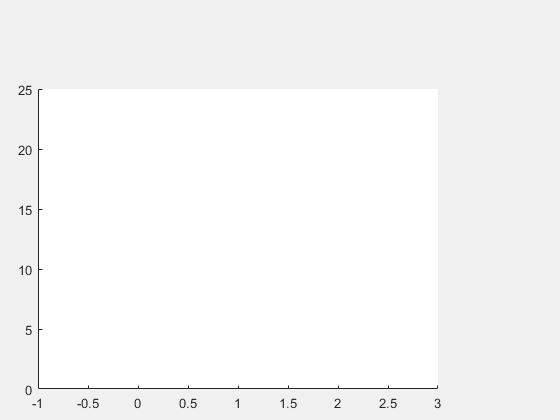Input Arguments

collapse all

Function returning graphics objects, specified as a function handle. For more information about function handles, see Create Function Handle.

Further arguments, specified as input arguments of a function handle that returns the graphics objects.

Name-Value Arguments

Specify optional comma-separated pairs of Name,Value arguments. Name is the argument name and Value is the corresponding value. Name must appear inside quotes. You can specify several name and value pair arguments in any order as Name1,Value1,...,NameN,ValueN.

Example: 'AnimationRange',[2 8],'FrameRate',30

Animation time parameter, specified as a symbolic variable.

Example: sym('y')

Range of the animation time parameter, specified as a two-element row vector. The two elements must be real values that are increasing.

Example: [-2 4.5]

Frame rate, specified as a positive value. The frame rate defines the number of frames per unit time for the animation object.

Example: 30

Output Arguments

collapse all

Animation object, returned as a scalar. You can use this object to query and modify the properties of the generated animation frames. For a list of properties, see Animator Properties.

Tips

• When you create a graph by using a plotting function, such as fplot, MATLAB® creates a series of graphics objects. You can then animate a specific property of the graphics objects by using the fanimator and the playAnimation functions. Note that some functions, such as title and xlabel, create text objects that cannot be animated. Instead, use the text function to create text objects that can be animated.